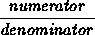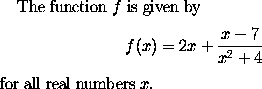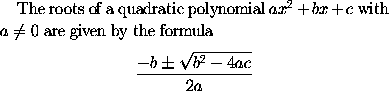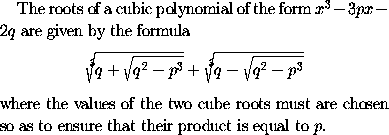### Fractions and Roots

Fractions of the formare obtained in LaTeX using the construction
\frac{numerator}{denominator}.
For example, to obtainone would type
The function $f$ is given by
$f(x) = 2x + \frac{x - 7}{x^2 + 4}$
for all real numbers $x$.


To obtain square roots one uses the control sequence

\sqrt{expression}.
For example, to obtainone would type
The roots of a quadratic polynomial $a x^2 + bx + c$ with
$a \neq 0$ are given by the formula
$\frac{-b \pm \sqrt{b^2 - 4ac}}{2a}$


In LaTeX, an nth root is produced using

\sqrt[n]{expression}.
For example, to obtainin LaTeX, one would type
The roots of a cubic polynomial of the form $x^3 - 3px - 2q$
are given by the formula
$\sqrt{q + \sqrt{ q^2 - p^3 }} + \sqrt{q - \sqrt{ q^2 - p^3 }}$
where the values of the two cube roots must are chosen
so as to ensure that their product is equal to $p$.